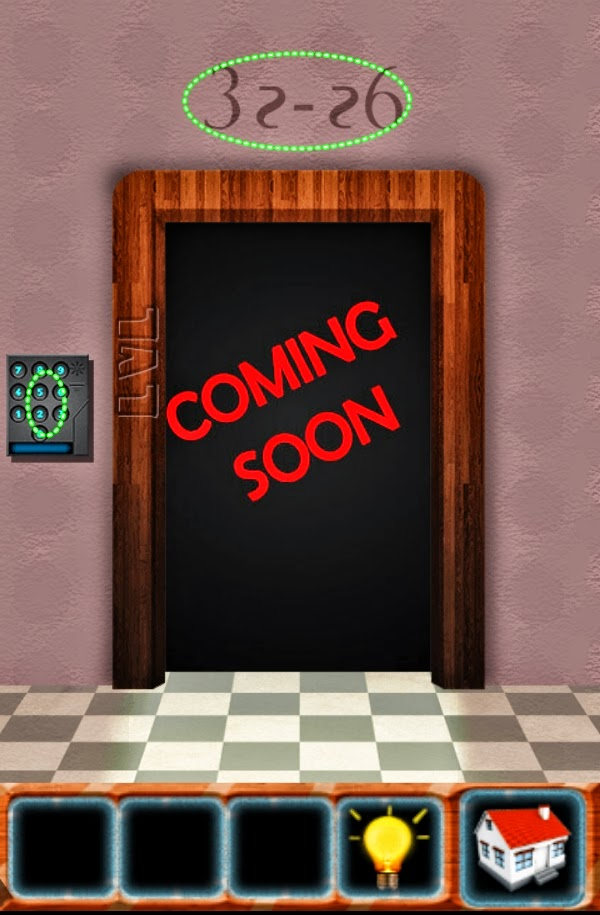100 Doors Classic Escape Level 56 57 58 59 60

# 100 Doors Classic Escape Level 56 57 58 59 60

100 Doors Classic Escape Level 56 Guide
Tap each number that changes color as the color sequence at number 9 on the top of the wall, and a number of colored number is 9
1 and 8 = black
2 and 7 = pink
3 and 6 = blue
4 and 5 = red## 100 Doors Classic Escape Level 57 Cheats

100 Doors Classic Escape Level 57 Walkthrough
See the sequence of forms at the top of the door
Tap each of the forms on the walls and in the doors
Top left circle, star left, right star, triangle top right
Middle left circle, star left, right star, middle right triangle
Lower left circle, star left, right star, triangle top right
Lower left circle, star left, lower right triangle

Solution 100 Doors Classic Escape Level 58
See the figures in the triangle, star, and square
Add up each number corresponding triangular shape, star, square
Star = 9 + 6 + 4 + 0 + 5 = 24
Triangle = 6 + 0 + 5 = 11
Square = 9 + 4 + 0 + 5 = 18
Tap the keypad and press the number 241118

### Walkthrough 100 Doors Classic Escape Level 59

What is the date and month of Valentine's Day
Tap the keypad and press the number 1402100 Doors Classic Escape Level 60 Guide

Guide 100 Doors Classic Escape Level 60
See the figure at the top of the wall as a guide
subtract the code of level 32 and 26
Code level 32 = 32 065
Code level 26 = 6212
32065-6212 = 25853
Tap the keypad and press the number 25853
Next to Level 61 62 63 64 65 on 100 Doors Classic Escape Solution